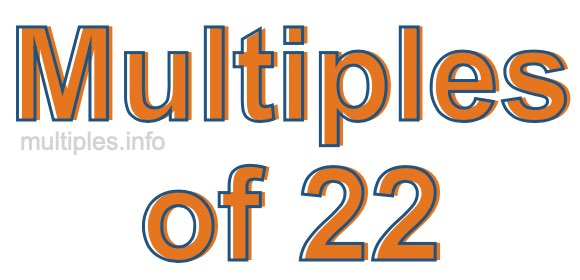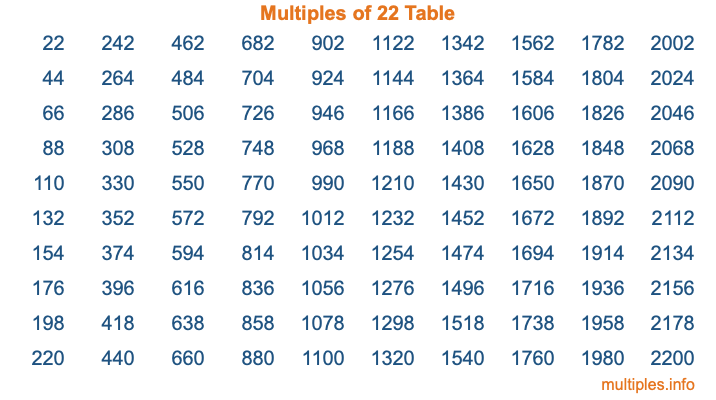Multiples of 22Welcome to the Multiples of 22 page. Here we will first teach you everything you will ever need to know about the multiples of 22, and then give you a study guide summary of everything we taught you to make sure you remember it all. Use this page to look up facts and learn information about the multiples of 22. This page will make you a multiples of twenty-two expert!

Definition of Multiples of 22
Multiples of 22 are all the numbers that when divided by 22 equal an integer. Each of the multiples of 22 are called a multiple. A multiple of 22 is created by multiplying 22 by an integer.

Therefore, to create a list of multiples of 22, you start with 1 multiplied by 22, then 2 multiplied by 22, then 3 multiplied by 22, and so on for as long as you want. Thus, the list of the first five multiples of 22 is 22, 44, 66, 88, and 110. To see a larger list of multiples of 22, see the printable image of Multiples of 22 further down on this page. We also have a category where you can choose any nth multiple of 22.

Multiples of 22 Checker
The Multiples of 22 Checker below checks to see if any number of your choice is a multiple of 22. In other words, it checks to see if there is any number (integer) that when multiplied by 22 will equal your number. To do that, we divide your number by 22. If the the quotient is an integer, then your number is a multiple of 22.

Is  a multiple of 22?

Least Common Multiple of 22 and ...
A Least Common Multiple (LCM) is the lowest multiple that two or more numbers have in common. This is also called the smallest common multiple or lowest common multiple and is useful to know when you are adding our subtracting fractions. Enter one or more numbers below (22 is already entered) to find the LCM.

Check out our LCM Calculator if you need more details about the Least Common Multiple or if you need the LCM for different numbers for adding and subtraction fractions.

nth Multiple of 22
As we stated above, 22 is the first multiple of 22, 44 is the second multiple of 22, 66 is the third multiple of 22, and so on. Enter a number below to find the nth multiple of 22.

th multiple of 22

Multiples of 22 vs Factors of 22
22 is a multiple of 22 and a factor of 22, but that is where the similarities end. All postive multiples of 22 are 22 or greater than 22. All positive factors of 22 are 22 or less than 22.

Below is the beginning list of multiples of 22 and the factors of 22 so you can compare:

Multiples of 22: 22, 44, 66, 88, 110, etc.

Factors of 22: 1, 2, 11, 22

As you can see, the multiples of 22 are all the numbers that you can divide by 22 to get a whole number. The factors of 22, on the other hand, are all the whole numbers that you can multiply by another whole number to get 22.

It's also interesting to note that if a number (x) is a factor of 22, then 22 will also be a multiple of that number (x).

Multiples of 22 vs Divisors of 22
The divisors of 22 are all the integers that 22 can be divided by evenly. Below is a list of the divisors of 22.

Divisors of 22: 1, 2, 11, 22

The interesting thing to note here is that if you take any multiple of 22 and divide it by a divisor of 22, you will see that the quotient is an integer.

Multiples of 22 Table
Below is an image of the first 100 multiples of 22 in a table. The table is in chronological order, column by column. The first column has the first ten multiples of 22, the second column has the next ten multiples of 22, and so on.The Multiples of 22 Table is also referred to as the 22 Times Table or Times Table of 22. You are welcome to print out our table for your studies.

Negative Multiples of 22
Although not often discussed or needed in math, it is worth mentioning that you can make a list of negative multiples of 22 by multiplying 22 by -1, then by -2, then by -3, and so on, to get the following list of negative multiples of 22:

-22, -44, -66, -88, -110, etc.

Multiples of 22 Summary
Below is a summary of important Multiples of 22 facts that we have discussed on this page. To retain the knowledge on this page, we recommend that you read through the summary and explain to yourself or a study partner why they hold true.

There are an infinite number of multiples of 22.

A multiple of 22 divided by 22 will equal a whole number.

22 divided by a factor of 22 equals a divisor of 22.

The nth multiple of 22 is n times 22.

The largest factor of 22 is equal to the first positive multiple of 22.

22 is a multiple of every factor of 22.

22 is a multiple of 22.

A multiple of 22 divided by a divisor of 22 equals an integer.

22 divided by a divisor of 22 equals a factor of 22.

Any integer times 22 will equal a multiple of 22.

Multiples of a Number
Here you can get the multiples of another number, all with the same attention to detail as we did for multiples of 22 on this page.

Multiples of
Multiples of 23
Did you find our page about multiples of twenty-two educational? Do you want more knowledge? Check out the multiples of the next number on our list!